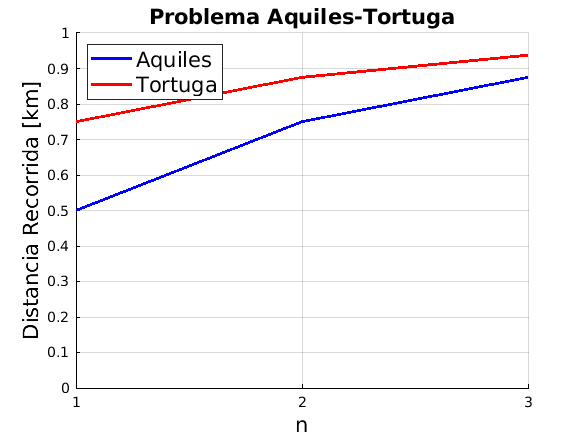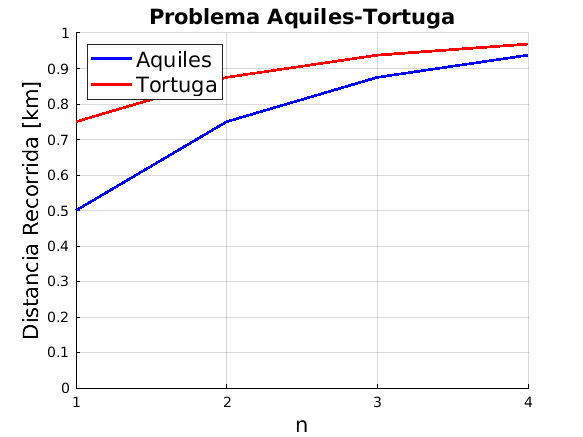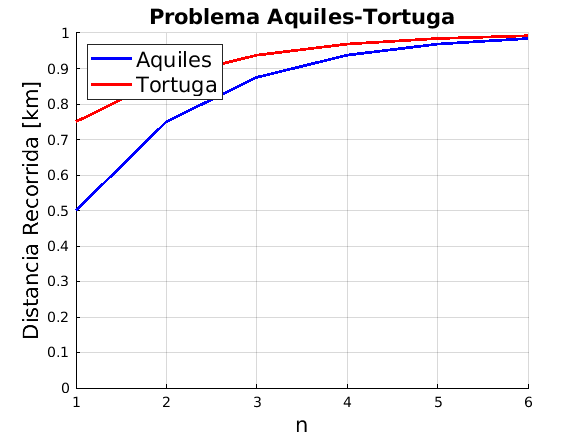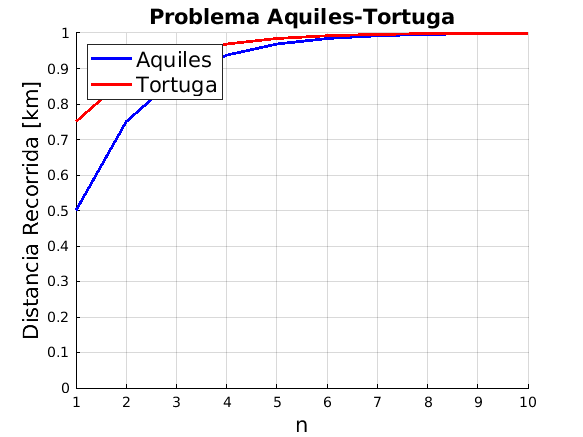Cody

# Problem 45248. Aquiles y la tortuga

Solution 2087618

Submitted on 10 Jan 2020 by Alejandro Sánchez Roncero
This solution is locked. To view this solution, you need to provide a solution of the same size or smaller.

### Test Suite

Test Status Code Input and Output
1   Pass
n = 3; suma = [1/2 (1/2+1/4) (1/2+1/4+1/8)]; assert(isequal(mi_funcion(n),suma));

tit = Text (Problema Aquiles-Tortuga) with properties: String: 'Problema Aquiles-Tortuga' FontSize: 11 FontWeight: 'bold' FontName: 'Helvetica' Color: [0 0 0] HorizontalAlignment: 'center' Position: [0.5000 1.0077 0.5000] Units: 'data' Show all properties leg = Legend (Aquiles, Tortuga) with properties: String: {'Aquiles' 'Tortuga'} Location: 'northeast' Orientation: 'vertical' FontSize: 9 Position: [0.7122 0.8201 0.1744 0.0789] Units: 'normalized' Show all properties

``
2   Pass
n = 4; suma = [1/2 (1/2+1/4) (1/2+1/4+1/8) (1/2+1/4+1/8+1/16)]; assert(isequal(mi_funcion(n),suma))

tit = Text (Problema Aquiles-Tortuga) with properties: String: 'Problema Aquiles-Tortuga' FontSize: 15 FontWeight: 'bold' FontName: 'Helvetica' Color: [0 0 0] HorizontalAlignment: 'center' Position: [2.0000 1.0106 0] Units: 'data' Show all properties leg = Legend (Aquiles, Tortuga) with properties: String: {'Aquiles' 'Tortuga'} Location: 'northwest' Orientation: 'vertical' FontSize: 15 Position: [0.1494 0.7743 0.2310 0.1247] Units: 'normalized' Show all properties

``
3   Pass
n = 6; suma = [1/2 (1/2+1/4) (1/2+1/4+1/8) (1/2+1/4+1/8+1/16) (1/2+1/4+1/8+1/16+1/32) (1/2+1/4+1/8+1/16+1/32+1/64)]; assert(isequal(mi_funcion(n),suma))

tit = Text (Problema Aquiles-Tortuga) with properties: String: 'Problema Aquiles-Tortuga' FontSize: 15 FontWeight: 'bold' FontName: 'Helvetica' Color: [0 0 0] HorizontalAlignment: 'center' Position: [2.5000 1.0106 0] Units: 'data' Show all properties leg = Legend (Aquiles, Tortuga) with properties: String: {'Aquiles' 'Tortuga'} Location: 'northwest' Orientation: 'vertical' FontSize: 15 Position: [0.1494 0.7743 0.2310 0.1247] Units: 'normalized' Show all properties

``
4   Pass
n = 10; suma = [1/2 (1/2+1/4) (1/2+1/4+1/8) (1/2+1/4+1/8+1/16) (1/2+1/4+1/8+1/16+1/32) (1/2+1/4+1/8+1/16+1/32+1/64) (1/2+1/4+1/8+1/16+1/32+1/64+1/2^7) (1/2+1/4+1/8+1/16+1/32+1/64+1/2^7+1/2^8) (1/2+1/4+1/8+1/16+1/32+1/64+1/2^7+1/2^8+1/2^9) (1/2+1/4+1/8+1/16+1/32+1/64+1/2^7+1/2^8+1/2^9+1/2^10)]; assert(isequal(mi_funcion(n),suma))

tit = Text (Problema Aquiles-Tortuga) with properties: String: 'Problema Aquiles-Tortuga' FontSize: 15 FontWeight: 'bold' FontName: 'Helvetica' Color: [0 0 0] HorizontalAlignment: 'center' Position: [3.5000 1.0106 0] Units: 'data' Show all properties leg = Legend (Aquiles, Tortuga) with properties: String: {'Aquiles' 'Tortuga'} Location: 'northwest' Orientation: 'vertical' FontSize: 15 Position: [0.1494 0.7743 0.2310 0.1247] Units: 'normalized' Show all properties

``

### Community Treasure Hunt

Find the treasures in MATLAB Central and discover how the community can help you!

Start Hunting!